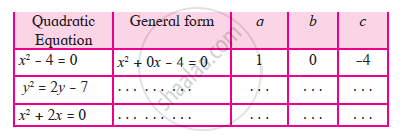Share

description

•  Introduction and Standard Form of a Quadratic Equation - ax2 + bx + c = 0, (a ≠ 0)

definition

The equation involving one variable and having 2 as the maximum index of the variable is called the quadratic equation.
General form is ax2 + bx + c = 0

notes

A quadratic equation in the variable x is an equation of the form ax^2 + bx + c = 0, where a, b, c are real numbers, a ≠ 0. For example, 2x^2 + x – 300 = 0 is a quadratic equation. Similarly, 2x^2 – 3x + 1 = 0, 4x – 3x^2 + 2 = 0 and 1 – x^2 + 300 = 0 are also quadratic equations.
In fact, any equation of the form p(x) = 0, where p(x) is a polynomial of degree 2, is a quadratic equation. But when we write the terms of p(x) in descending order of their degrees, then we get the standard form of the equation. That is, ax^2 + bx + c = 0, a ≠ 0 is called the standard form of a quadratic equation.
Standard form of quadratic equation.
The equation involving one variable and having 2 as the maximum index of the variable is called the quadratic equation.
General form is ax^2 + bx + c = 0
In ax^2 + bx + c = 0, a, b, c are real numbers and a≠ 0.
ax^2 + bx + c = 0 is the general form of quadratic equation.
Activity : Complete the following tableIn the equation 3x^2 - 5x + 3 = 0, x is the only variable and maximum index of the variable is 2
∴ It is a quadratic equation.

Shaalaa.com

Quadratic Equation part 1 (Introduction) [00:06:43]
S
1
0%

S